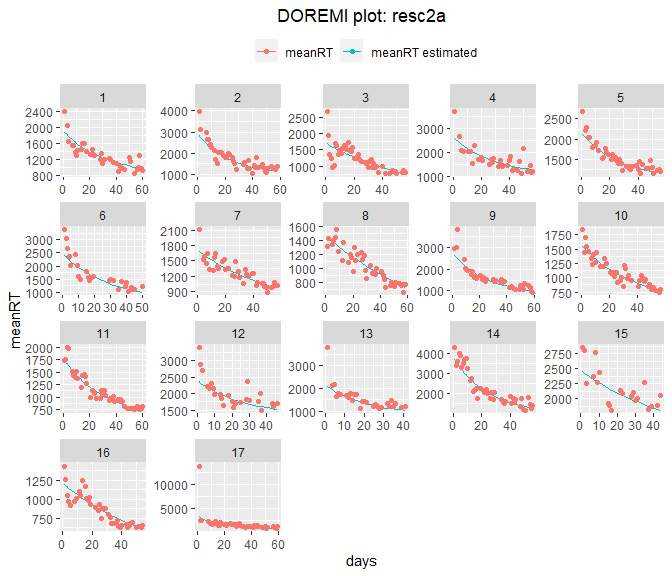# first-order

library(doremi)

# FIRST ORDER DIFFERENTIAL EQUATIONS

The linear first order differential equation with constant coefficients model is given by the following equation: $\tau\frac{dy}{dt} + (y-y_{eq}) = ku(t)$ or $\frac{dy}{dt} + \gamma (y-y_{eq}) = \gamma ku(t)$ with the initial condition: $y(t=0)=y_{0}$ Where:

• $$y(t)$$ is the signal to be analyzed

• $$\frac{dy}{dt}$$ is its first derivative

• $$u(t)$$ is the excitation term perturbing the dynamics of $$y(t)$$

And regarding the coefficients:

• $$\gamma$$ is the decay rate.Its inverse, $$\tau$$ represents the characteristic response time of the solution of the differential equation (i.e. the time it takes to the system to go back to equilibrium)

• $$k$$ is the gain. It indicates the proportionality between the stationary increase of $$y$$ and the constant excitation increase that caused it.

• $$yeq$$ is the signal equilibrium value. Value reached when the excitation term is 0.

It is common to find this equation with the excitation term set to 0, $$u(t)=0$$:

$\frac{dy}{dt} + \gamma(y-y_{eq}) = 0$

The dynamics in this case follow and exponential decay of the form $$y(t)=(y_0-yeq) e^{-\gamma t} + y_{eq}$$ and are provoked either by a previous excitation that is no longer present or by the displacement of the system from its equilibrium position (i.e. an initial condition different from 0)

# Two steps analysis procedure

The estimation of the fixed effect coefficients of the differential equation is performed in two steps. The first step consists in estimating the first derivative, using one of the three methods proposed.

The second step consists in performing the following linear mixed-effects regression:

$\begin{equation} \dot{y}_{ij} \sim (b_{0}+b_{0j})+ (b_1 + b_{1j})y_{ij}+ (b_2 + b_{2j}) U_{ij}+e_{ij} \label{eq2} \end{equation}$

where:

• i accounts for the time
• j accounts for the different individuals
• $$\dot{y}_{ij}$$ is the derivative estimated through one of the derivative estimation methods available in the package (gold, glla and fda) calculated on embedding points (gold, glla) or estimated on the time points provided (fda)
• $$y_{ij}$$ and $$U_{ij}$$ are the variable and the excitation averaged on embedding points (gold, glla) or the original values provided (fda)
• $$e_{ij}$$ is the error term (residuals)

Note that random effects are estimated for the intercept ($$b_{0} + b_{0j}$$), variable ($$b_{1} + b_{1j}$$) and excitation terms ($$b_{2} + b_{2j}$$), so that individuals can have different coefficients (initial condition, damping time, gain and equilibrium value).

The fixed effect coefficients estimated from the regression are:

• Coefficient $$gamma = b_{1}$$
• Coefficient $$yeqgamma = b_{0}$$
• Coefficient $kgamma = b_{2}$

The coefficients of the differential equation can thus be calculated as:

• Decay time: $$tau = \tau = \frac{1}{b_{1}}$$
• Gain: $$k = \frac{b_{2} }{b_{1}}$$
• Equilibrium value: $$yeq = \frac{b_{0} }{b_1}$$

The estimation is performed using the function lmer if there are several individuals or lm if there is a single individual.

# First order differential equation with no excitation term

## Simulating data

Two functions are available to simulate data in the package: generate.1order simulates the solution of the differential equation for a given vector of time, and the parameters tau = $$\tau$$, y0 = $$y(t=0)$$,yeq = $$y_{eq}$$, k = $$k$$ and the vector of excitation $$u(t)$$. The function create a data.table with a column t for the time, y for the signal, and exc for the excitation.

test <- generate.1order(0:100,y0 = 10,tau = 10)
plot(test$t,test$y)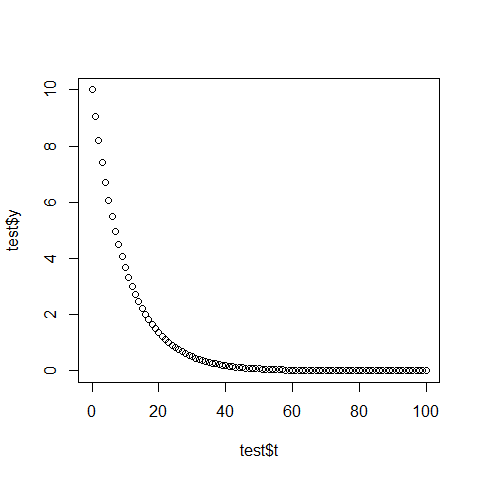The function generate.panel.1order uses generate.1order to generate a panel of nind individuals, with measurement noise and inter-individual noise.

### Example 1a - Generating signals with no noise

In this example we will generate data for 4 individuals, with a decay time of 10s. That is, the signal follows exactly the differential equation (no measurement noise) with no variation of the decay time, the gain and the equilibrium value across individuals (no interindividual noise). As we are considering no excitation, then, as mentioned, the system should be out of its equilibrium value to observe the dynamics of return to equilibrium.

res1a <- generate.panel.1order(time = 0:99,
y0 = 1,
tau = 10,
nind = 4)

It can be specified to the function whether there is an excitation or not by either setting the input to NULL or directly ignoring it in the input arguments. The function returns a data.table with the class ‘doremidata’. As any data.table, it can be visualized using the str or head functions or entering the variable name.

res1a
#>      id time    signalraw       signal
#>   1:  1    0 1.000000e+00 1.000000e+00
#>   2:  1    1 9.048378e-01 9.048378e-01
#>   3:  1    2 8.187322e-01 8.187322e-01
#>   4:  1    3 7.408199e-01 7.408199e-01
#>   5:  1    4 6.703215e-01 6.703215e-01
#>  ---
#> 396:  4   95 7.485298e-05 7.485298e-05
#> 397:  4   96 6.773005e-05 6.773005e-05
#> 398:  4   97 6.128494e-05 6.128494e-05
#> 399:  4   98 5.545313e-05 5.545313e-05
#> 400:  4   99 5.017628e-05 5.017628e-05

Where:

• id is the identifier of the individual

• time is the time vector introduced as input

• signalraw is the signal without noise

• signal is the signal with noise (in this case, the values of this column are the same as those of the signalraw column and in the plot, and thus both lines are overlapped in the plot below).

Plotting the data with the plot method available in the package for doremidata objects is quite straight forward:

plot(res1a)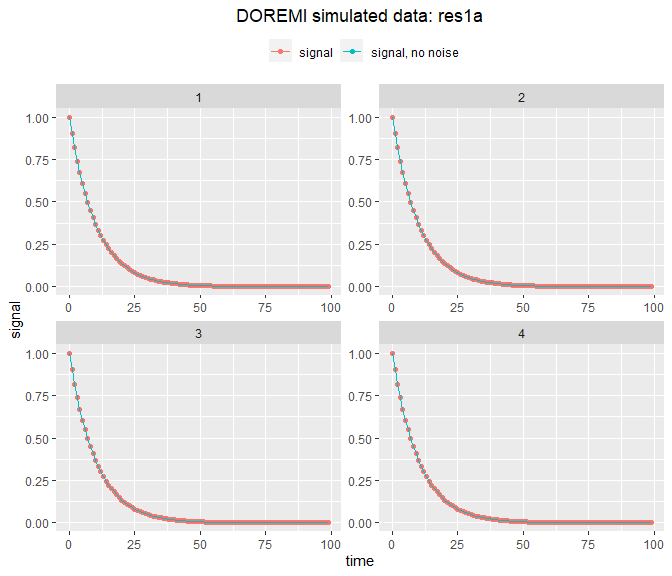### Example 1b - Changing initial condition, gain and equilibrium value

In this example, we show the flexibility of the functions to add changes on the mentioned parameters. For this, we simplify the form of the exitation to a simple step:

timevec <- 0:49
set.seed(123)
res1b <- generate.panel.1order(time = timevec,
excitation = as.numeric(timevec > 20),
y0 = 3,
tau = 5,
yeq = 1.5,
nind = 4)
plot(res1b) +
ggplot2::scale_y_continuous(limits = c(0, 3))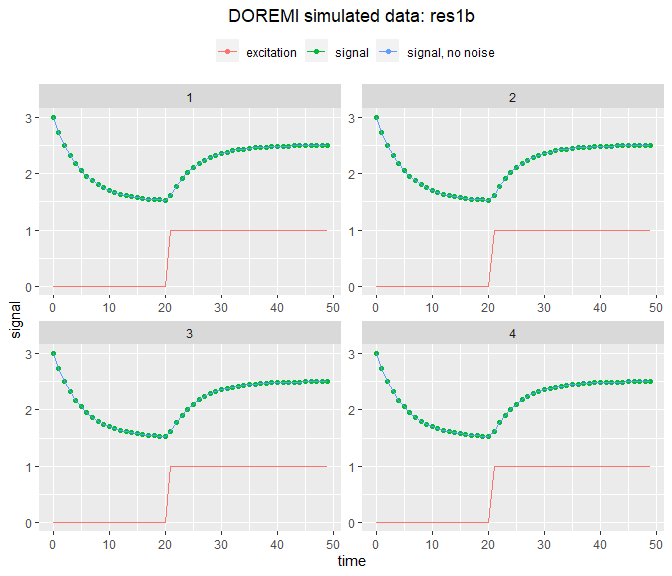### Example 2a - Generating signals with noise

The call to the function remains almost the same, with two additional arguments: a noise to signal ratio, set at 0.2, and an interindividual noise set at 40%. We can then visualize the different trajectories for the different individuals:

# Generation of signals with intra and inter-noise
set.seed(123)
res2a <- generate.panel.1order(time = 0:49,
y0 = 1,
tau = 5,
nind = 6,
internoise = 0.4,
intranoise = 0.1)
plot(res2a)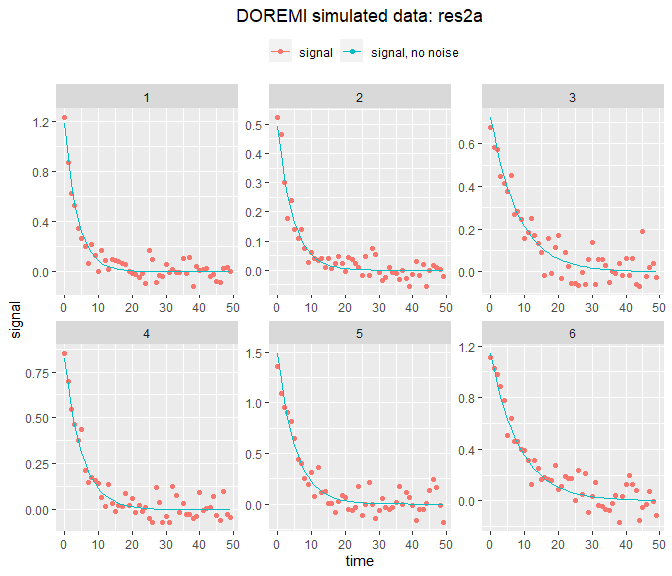## Analyzing data

### Example 3 - Analyzing data when the excitation is unknown, with some inter- and intraindividual noise

We will test how the analysis functions fit a decreasing exponential, as is the case when there is no excitation, when it is unknown, or when it is constant over time.

#Simulating data with these hypothesis
set.seed(123)
data3 <- generate.panel.1order(time = 0:50,
y0 = 0.5,
tau = 10,
nind = 3,
internoise = 0.2,
intranoise = 0.1)
#Analyzing

res3 <- analyze.1order(data = data3,
id = "id",
time = "time",
signal = "signal",
dermethod = "fda",
derparam = 0.7)
#> WARN [2021-01-19 14:27:57] No excitation signal introduced as input. Input was set to 0.

Note that the input parameter has been omitted when calling the function. The result display the summary of the fixed effect coefficients:

res3
#>     id      gamma  gamma_stde      yeqgamma yeqgamma_stde      tau         yeq
#> 1: All 0.08519334 0.008371114 -0.0009016283  0.0003712671 11.73801 -0.01058332
#>           R2
#> 1: 0.8072038

The data.table result_id gives the coefficients estimated for each individual:

excitation = U$exc, tau = 10, k = 1, nind = 4, yeq = 2, y0 = 2, internoise = 0.2, intranoise = 2) plot(res2a)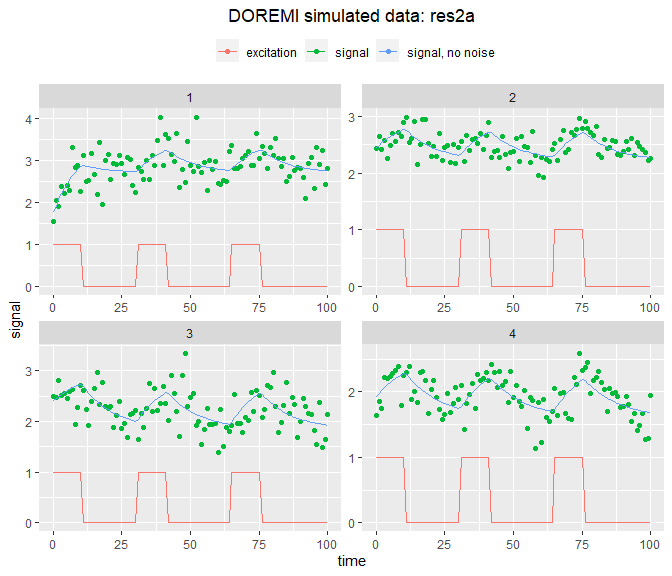# Analyzing data ## Example 3 - Analyzing data from a single individual In this case, we can reuse one of the signals from the previous example data3 <- res2a[id==1] As the table contains data from a single individual, the id parameter has been omitted when calling the function. #Analyzing res3 <- analyze.1order(data = data3, input = "excitation", time ="time", signal = "signal", verbose=T) #> INFO [2021-01-19 14:28:01] Input data contains single individual. #> INFO [2021-01-19 14:28:01] One or several excitations. Linear regression calculated #> INFO [2021-01-19 14:28:01] Linear mixed-effect model had no errors. #> INFO [2021-01-19 14:28:01] One or several excitation terms. Calculation of estimated signal with deSolve res3 #> id gamma gamma_stde yeqgamma yeqgamma_stde tau yeq #> 1: All 0.06526137 0.08681774 0.168252 0.2511207 15.323 2.578126 #> excitation_kgamma excitation_kgamma_stde excitation_k R2 #> 1: 0.08469348 0.05704505 1.297758 0.342613 #Plotting plot(res3)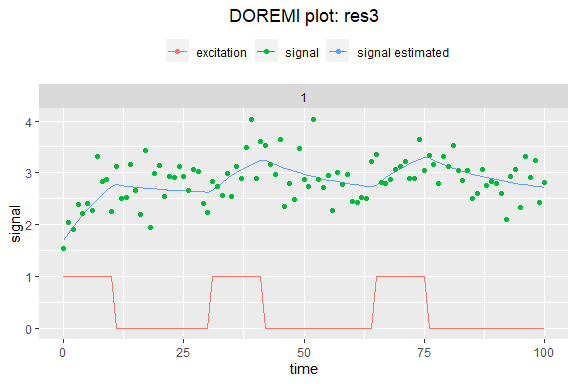## Example 3a - Analyzing data with several individuals and some inter and intra-individual noise Analyzing the data generated in the example 2 above (table res2a), the user must specify the name of the columns containing the id of the participants, the excitation, and the signal. As several methods are available for the estimation of the derivatives, the user needs to specify which method to use (gold is the default) and the embedding dimension by modifying the parameter derparam (see the package pdf manual for more details). res3a <- analyze.1order(data = res2a, id = "id", input ="excitation", time ="time", signal = "signal", dermethod = "gold", derparam = 3) Now let’s take a look at the result. When calling the variable, the fixed effect coefficients of the regression with their associated standard error, the derived coefficient characterizing the signal shape (the decay time tau, the equilibrium value yeq and the gain k), and the R2 are displayed: res3a #> id gamma gamma_stde yeqgamma yeqgamma_stde tau yeq #> 1: All 0.006561924 0.0239843 -0.006529568 0.05778508 152.3943 -0.9950691 #> excitation_kgamma excitation_kgamma_stde excitation_k R2 #> 1: 0.06712488 0.0227174 10.22945 0 The signal analyzed was generated with tau = 10, yeq = 0, and k = 1. This is a simplified view of the results. The analysis function supplies an object of class “doremi” that contains in fact several lists. It is possible to explore the full result values by using the function “summary” for doremi objects (see the section on methods created for doremi objects at the end of this vignette). summary(res3a) #> Derivative and mean calculation ($data):
#>      id excitation time   signal signal_rollmean signal_derivate1 time_derivate
#>   1:  1          1    0 1.548851        1.834129      0.181351993             1
#>   2:  1          1    1 2.041981        2.114180      0.173511265             2
#>   3:  1          1    2 1.911555        2.170934      0.150343864             3
#>   4:  1          1    3 2.389003        2.335365      0.007922849             4
#>   5:  1          1    4 2.212243        2.300707      0.036393743             5
#>  ---
#> 400:  4          0   96 1.487556        1.477797     -0.106773663            97
#> 401:  4          0   97 1.671826        1.412553     -0.189999930            98
#> 402:  4          0   98 1.274009        1.502412      0.333695804            99
#> 403:  4          0   99 1.291826              NA               NA            NA
#> 404:  4          0  100 1.941400              NA               NA            NA
#>      excitation_rollmean totalexc totalexcroll signal_estimated
#>   1:                   1 10.22945     10.22945         1.785409
#>   2:                   1 10.22945     10.22945         1.834129
#>   3:                   1 10.22945     10.22945         1.882530
#>   4:                   1 10.22945     10.22945         1.930615
#>   5:                   1 10.22945     10.22945         1.978385
#>  ---
#> 400:                   0  0.00000      0.00000         1.948274
#> 401:                   0  0.00000      0.00000         1.929023
#> 402:                   0  0.00000      0.00000         1.909898
#> 403:                  NA  0.00000           NA         1.890899
#> 404:                  NA  0.00000           NA         1.872023
#>
#>  Mixed-effects regression results ($regression): #> [] #> Linear mixed model fit by REML. t-tests use Satterthwaite's method [ #> lmerModLmerTest] #> Formula: paste0("signal_derivate1 ~ signal_rollmean + (1 +", paste(doremiexc, #> "rollmean ", collapse = "+", sep = "_"), " + signal_rollmean |id) + ", #> paste(doremiexc, "rollmean ", collapse = "+", sep = "_")) #> Data: intdata #> Control: lmerControl(calc.derivs = FALSE, optimizer = "nloptwrap") #> #> REML criterion at convergence: -138.8 #> #> Scaled residuals: #> Min 1Q Median 3Q Max #> -3.1701 -0.6832 -0.0177 0.6163 4.2287 #> #> Random effects: #> Groups Name Variance Std.Dev. Corr #> id (Intercept) 1.718e-09 4.145e-05 #> input1_rollmean 1.013e-09 3.183e-05 -0.26 #> signal_rollmean 2.207e-10 1.486e-05 -0.99 0.10 #> Residual 3.963e-02 1.991e-01 #> Number of obs: 396, groups: id, 4 #> #> Fixed effects: #> Estimate Std. Error df t value Pr(>|t|) #> (Intercept) -0.006530 0.057785 392.960102 -0.113 0.91009 #> signal_rollmean -0.006562 0.023984 392.971440 -0.274 0.78454 #> input1_rollmean 0.067125 0.022717 392.940144 2.955 0.00332 ** #> --- #> Signif. codes: 0 '***' 0.001 '**' 0.01 '*' 0.05 '.' 0.1 ' ' 1 #> #> Correlation of Fixed Effects: #> (Intr) sgnl_r #> signl_rllmn -0.977 #> inpt1_rllmn -0.018 -0.110 #> #> [] #>$id
#>     (Intercept) input1_rollmean signal_rollmean
#> 1  4.579499e-10    1.196365e-08   -1.078989e-09
#> 2  3.937595e-09   -7.368405e-09   -8.931788e-10
#> 3 -1.325579e-10   -9.584872e-09    7.779417e-10
#> 4 -4.262987e-09    4.989627e-09    1.194226e-09
#>
#> with conditional variances for "id"
#>
#>
#>  Mean coefficients of the differential equation ($resultmean): #> id gamma gamma_stde yeqgamma yeqgamma_stde tau yeq #> 1: All 0.006561924 0.0239843 -0.006529568 0.05778508 152.3943 -0.9950691 #> excitation_kgamma excitation_kgamma_stde excitation_k R2 #> 1: 0.06712488 0.0227174 10.22945 0 #> #> Coefficients per individual ($resultid):
#>    id       gamma     yeqgamma      tau        yeq excitation_kgamma
#> 1:  1 0.006561923 -0.006529568 152.3943 -0.9950689        0.06712489
#> 2:  2 0.006561924 -0.006529564 152.3943 -0.9950684        0.06712487
#> 3:  3 0.006561925 -0.006529568 152.3943 -0.9950692        0.06712487
#> 4:  4 0.006561926 -0.006529573 152.3943 -0.9950699        0.06712488
#>    excitation_k
#> 1:     10.22945
#> 2:     10.22945
#> 3:     10.22945
#> 4:     10.22945
#>
#>  Derivative estimation method used ($dermethod): #>  "calculate.gold" #> #> Embedding number/smoothing parameter ($derparam):
#>  3

The first object of the output contains the original data with some columns added. These columns contain intermediate variables necessary for the mixed-effect regression:

head(res3a$data) #> id excitation time signal signal_rollmean signal_derivate1 time_derivate #> 1: 1 1 0 1.548851 1.834129 0.181351993 1 #> 2: 1 1 1 2.041981 2.114180 0.173511265 2 #> 3: 1 1 2 1.911555 2.170934 0.150343864 3 #> 4: 1 1 3 2.389003 2.335365 0.007922849 4 #> 5: 1 1 4 2.212243 2.300707 0.036393743 5 #> 6: 1 1 5 2.404849 2.666189 0.451919842 6 #> excitation_rollmean totalexc totalexcroll signal_estimated #> 1: 1 10.22945 10.22945 1.785409 #> 2: 1 10.22945 10.22945 1.834129 #> 3: 1 10.22945 10.22945 1.882530 #> 4: 1 10.22945 10.22945 1.930615 #> 5: 1 10.22945 10.22945 1.978385 #> 6: 1 10.22945 10.22945 2.025842 Where: • signal_rollmean contains the zeroth order derivative of the input signal in embedding points provided by gold or glla, or the smoothing performed by fda. • signal_derivate1 contains the first derivate of signal, calculated by using one of the derivative estimation methods available (gold, glla, fda). • time_derivate contains the values of time in which the derivative was evaluated. • excitation_rollmean contains the roll mean of the excitation signal in embedding points (gold, glla) or in the initial time points provided (fda). If we want to visualize the summary of the mixed-effect regression: res3a$regression
#> []
#> Linear mixed model fit by REML. t-tests use Satterthwaite's method [
#> lmerModLmerTest]
#> Formula: paste0("signal_derivate1 ~ signal_rollmean + (1 +", paste(doremiexc,
#>     "rollmean ", collapse = "+", sep = "_"), " + signal_rollmean |id) + ",
#>     paste(doremiexc, "rollmean ", collapse = "+", sep = "_"))
#>    Data: intdata
#> Control: lmerControl(calc.derivs = FALSE, optimizer = "nloptwrap")
#>
#> REML criterion at convergence: -138.8
#>
#> Scaled residuals:
#>     Min      1Q  Median      3Q     Max
#> -3.1701 -0.6832 -0.0177  0.6163  4.2287
#>
#> Random effects:
#>  Groups   Name            Variance  Std.Dev.  Corr
#>  id       (Intercept)     1.718e-09 4.145e-05
#>           input1_rollmean 1.013e-09 3.183e-05 -0.26
#>           signal_rollmean 2.207e-10 1.486e-05 -0.99  0.10
#>  Residual                 3.963e-02 1.991e-01
#> Number of obs: 396, groups:  id, 4
#>
#> Fixed effects:
#>                   Estimate Std. Error         df t value Pr(>|t|)
#> (Intercept)      -0.006530   0.057785 392.960102  -0.113  0.91009
#> signal_rollmean  -0.006562   0.023984 392.971440  -0.274  0.78454
#> input1_rollmean   0.067125   0.022717 392.940144   2.955  0.00332 **
#> ---
#> Signif. codes:  0 '***' 0.001 '**' 0.01 '*' 0.05 '.' 0.1 ' ' 1
#>
#> Correlation of Fixed Effects:
#>             (Intr) sgnl_r
#> signl_rllmn -0.977
#> inpt1_rllmn -0.018 -0.110
#>
#> []
#> $id #> (Intercept) input1_rollmean signal_rollmean #> 1 4.579499e-10 1.196365e-08 -1.078989e-09 #> 2 3.937595e-09 -7.368405e-09 -8.931788e-10 #> 3 -1.325579e-10 -9.584872e-09 7.779417e-10 #> 4 -4.262987e-09 4.989627e-09 1.194226e-09 #> #> with conditional variances for "id" Where we have the random and fixed effects and the residuals calculated by the function lmer or lm depending on if the sample had several or one individual respectively. Beware that, as known in most two-steps procedures, the estimation of derivatives reduces variance, and thus the error terms provided by the regression are not final. The following table contains the fixed effect coefficients (result displayed by default when calling a doremi variable, as mentioned before): res3a$resultmean
#>     id       gamma gamma_stde     yeqgamma yeqgamma_stde      tau        yeq
#> 1: All 0.006561924  0.0239843 -0.006529568    0.05778508 152.3943 -0.9950691
#>    excitation_kgamma excitation_kgamma_stde excitation_k R2
#> 1:        0.06712488              0.0227174     10.22945  0

It can be observed that the decay time is close to the value introduced to the simulation function (6), the equilibrium value close to its true value (0), and the gain close to its true value (1). For each individual we have:

res3a$resultid #> id gamma yeqgamma tau yeq excitation_kgamma #> 1: 1 0.006561923 -0.006529568 152.3943 -0.9950689 0.06712489 #> 2: 2 0.006561924 -0.006529564 152.3943 -0.9950684 0.06712487 #> 3: 3 0.006561925 -0.006529568 152.3943 -0.9950692 0.06712487 #> 4: 4 0.006561926 -0.006529573 152.3943 -0.9950699 0.06712488 #> excitation_k #> 1: 10.22945 #> 2: 10.22945 #> 3: 10.22945 #> 4: 10.22945 Where: • tau is the decay time. • yeq is the equilibrium value. • excitation_k is the gain associated to the excitation “excitation”. As we will see later, there is the possibility to consider several excitations, each one of which will have a different effect on the dynamics of the signal studied and thus, a different gain associated to them. If we graphically wish to verify how the estimated signal fits the initial signal, we can once again call the function plot, that has been adapted to handle doremi objects as well: plot(res3a)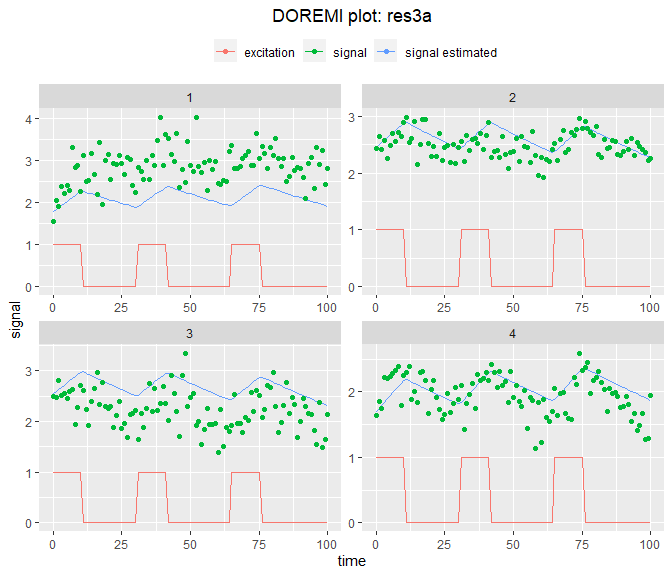Similarly to the print function, plot applied to a doremi object plots by default the first six individuals contained in the result. If we wish to visualize a single individual or a specific set of individuals, we can specify them by changing the “id” input parameter of the function: plot(res3a, id = 3)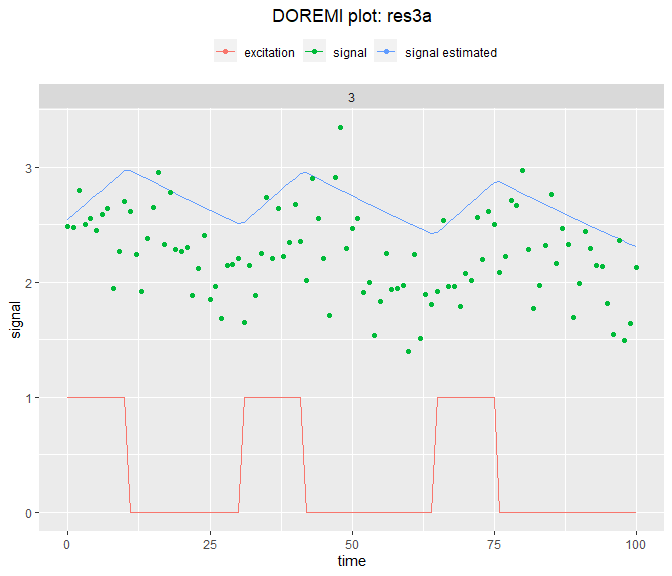plot(res3a, id = c(1,4))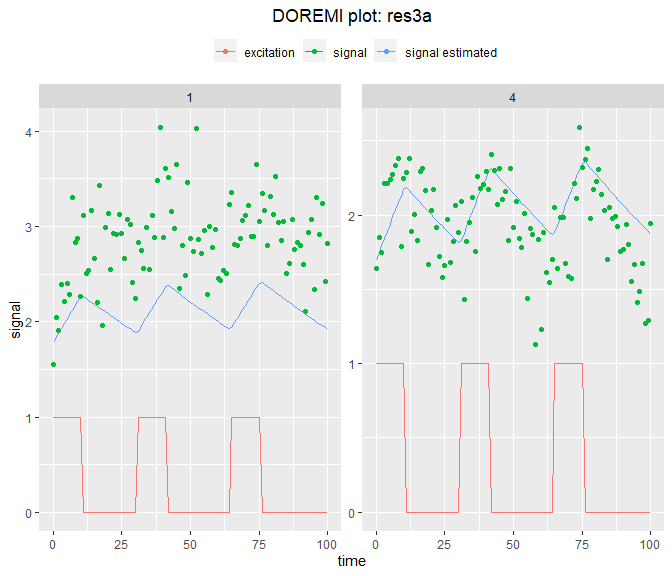Finally, the results contain the derivative method used and the embedding number/smoothing parameter used for further reference. ## Example 3b - Enhancing the fit by changing the embedding number/ smoothing parameter. As mentioned before, the estimation of derivatives before model fit is a source of error underestimation. It is possible to enhance the quality of the fit in three ways: • By changing the embedding number/smoothing parameter • By changing the order of the derivative • By changing the derivation method In the following example, we will use the function optimum_param to find the embedding number that provides the closest $$R^2$$ to 1. The other ways can be tested “manually” by calling the other functions, for instance, in a simulation study. Let us reuse the data from res2a: res3b <- optimum_param (data = res2a, id = "id", input = "excitation", time = "time", signal = "signal", model = "1order", dermethod = "gold", pmin = 3, pmax = 21, pstep = 2) res3b$summary_opt
res3b$d #>  13 And can be seen that from the range provided, an embedding number of {r} res3b$d produces the best fit and the coefficients are closer to their true values than the ones estimated in the previous example.

The optimum_param function generates an object of class “doremiparam”. If we want to graphically see the evolution of the coefficients according to the embedding number, we can easily plot the results with the plot function too:

plot(res3b)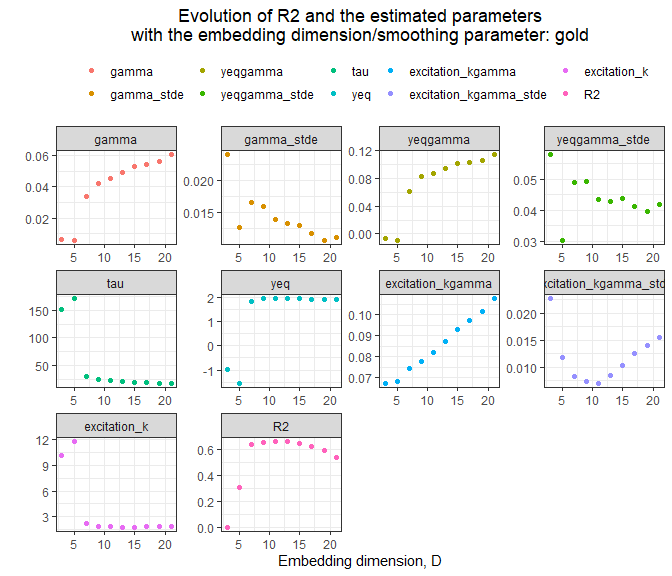## Example 4 - Analyzing data when the signal is subject to several excitations

In this example, we construct a signal having different gain for different excitation sources. For the sake of simplicity, we generate this example signal without noise.

#Simulating data with these hypothesis
#Generating the three excitation signals:
t <- 0:100
u1 <- as.numeric(t>20 & t<40)
u2 <- as.numeric(t>50 & t<70)
u3 <- as.numeric(t>80 & t<100)
# Arbitrarily choosing a = 1, b = 2 and c = 5 for the first individual
et1 <- u1 + 3 * u2 + 5 * u3
y1 <- generate.1order(time = t,
excitation = et1,
tau = 10,
k = 1)$y #as we are using the$y argument of the object generated

#Signals for the second individual;
# Arbitrarily choosing a = 1, b = 2.5 and c = 4 for the second individual
et2 <- u1 + 2.5 * u2 + 4 * u3
y2 <- generate.1order(time = t,
excitation = et2,
tau = 10,
k = 1)$y #Generating a table with the signals data4 <- data.frame(id = rep(c(1, 2), c(length(et1), length(et2))), time = c(t, t), excitation1 = c(u1, u1), excitation2 = c(u2, u2), excitation3 = c(u3, u3), signalcol = c(y1, y2)) We have in data4 a signal, whose dynamics was created by three excitations with different gains: k = 1, 3 and 5 for id = 1, k = 1, 2.5 and 4 for id = 2. We can see in the graph below that each excitation is identical but generates a different change of the signal: #Plotting signals ggplot2::ggplot( data = data4) + ggplot2::geom_line(ggplot2::aes(time,signalcol, colour = "Signal-no noise"))+ ggplot2::geom_line(ggplot2::aes(time,excitation1,colour = "excitation 1"))+ ggplot2::geom_line(ggplot2::aes(time,excitation2,colour = "excitation 2"))+ ggplot2::geom_line(ggplot2::aes(time,excitation3,colour = "excitation 3"))+ ggplot2::facet_wrap(~id)+ ggplot2::labs(x = "Time (s)", y = "Signal (arb. unit)", colour = "")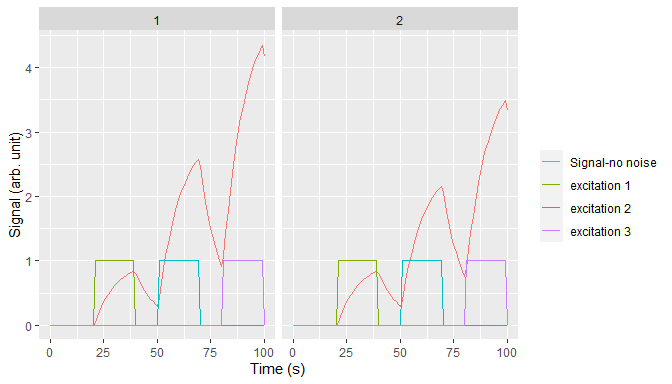It is possible in analyze.1order to give a vector of column as input. In this case, a gain will be estimated for each excitation column. #Analyzing signals res4 <- analyze.1order(data = data4, id = "id", input = c("excitation1", "excitation2", "excitation3"), time = "time", signal = "signalcol", dermethod = "fda", derparam = 0.1) #Looking for the calculation of the coefficients of the excitation res4$resultid

Here, we recover the estimated gains for each individual in the resultid table. They are a good approximation of the coefficients introduced, using this small sample of 2 individuals.

#Plotting signals
plot(res4)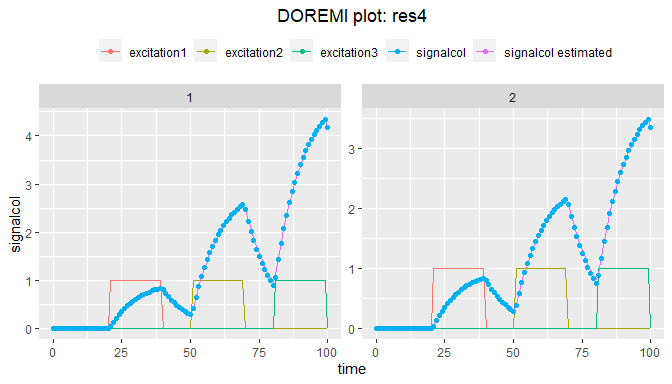## Example 5 - Analyzing data with missing values in the signal

We examine how the analysis function still manages to retrieve an accurate fit from signals with missing measurements:

t <- 0:200
set.seed(123)
data5 <- generate.panel.1order(time = t,
excitation = as.numeric(t>50 & t<100),
tau = 10,
k = 1,
nind = 6,
internoise = 0.4,
intranoise = 0.1)
plot(data5)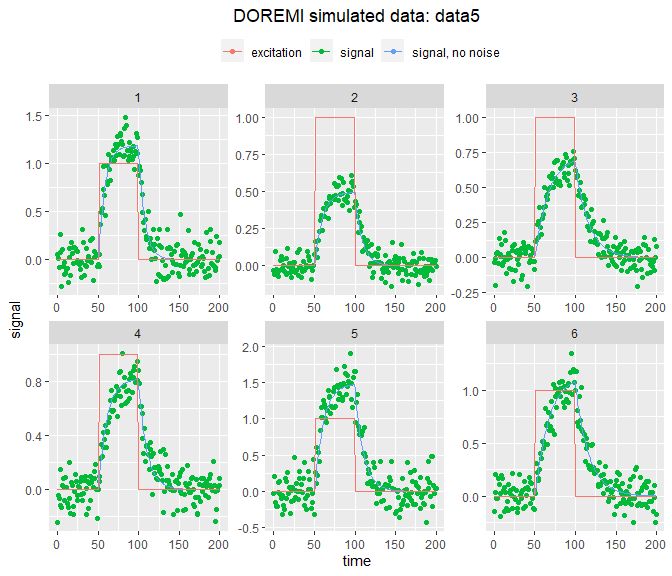#Keeping one third of the rows selected randomly from the full data set
set.seed(123)
data5rd <- data5[sample(nrow(data5), nrow(data5)/3), ]
data5rd <- data5rd[order(id,time)]

In the next plot we show the selection of random points made on the full data set, and also represent the signal without noise. The signal with noise will be used to carry out the analysis.

plot(data5rd)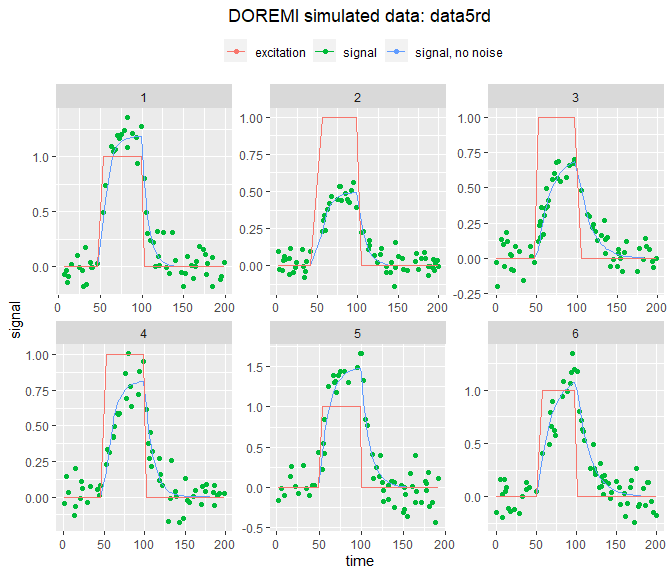Then analyzing and visualizing the random points selection:

res7 <- analyze.1order(data = data5rd,
id = "id",
input = "excitation",
time ="time",
signal = "signal")
plot(res7)+
ggplot2::geom_line(data = data5,
ggplot2::aes(time,signalraw, colour = "Original signal"))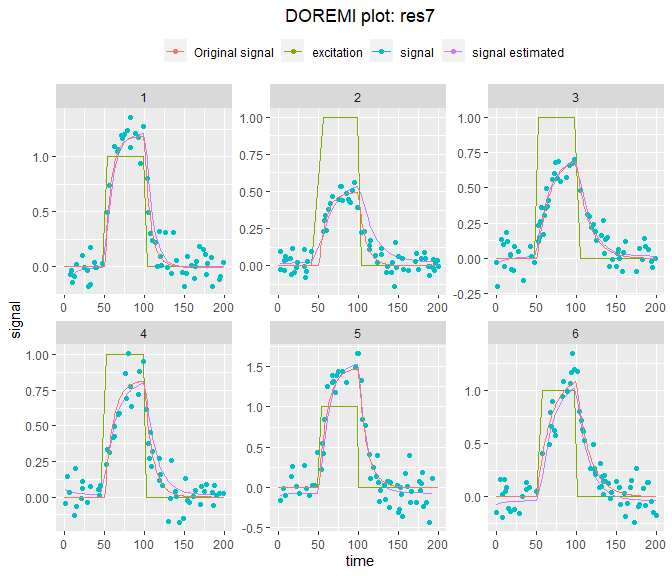# C - Applications

## Heart rate during effort tests

Using the cardio dataset present in this package, we will apply the model to real heart frequency measurements. The data fields are described with detail in the pdf manual of the package, together with the source from which the data was obtained. The data consists in heart rate measurements from 21 individuals carrying out an effort test on a cycle ergometer. Participants pedal on a bicycle with increasing resistance. The resistance load is measured in watts, with higher load forcing the individual to greater effort. His/her heart frequency will then vary according to the effort supplied and the participant’s fitness. According to this, the cardio data includes, for each individual, the time since the beginning of the test (seconds), the bicycle load (Watts) and the heart rate (1/min). Here is an example of these data:

ggplot2::ggplot(cardio[id == 2],
ggplot2::aes(x = time))+
ggplot2::geom_point(ggplot2::aes(y = hr,color = "heart rate (beat/s)"))+
ggplot2::geom_line(ggplot2::aes(y = load,color = "effort load (W)"))

We can perform the analysis using analyze.1order:

resc1a <- analyze.1order(data = cardio,
id = "id",
time ="time",
signal = "hr",
dermethod = "glla",
derparam = 5)

And use the plot function to plot the analysis results for all 21 participants. Note that omitting the argument id would lead to only the first 6 participants being plotted by default.

plot(resc1a, id = 1:21) +
ggplot2::facet_wrap(~id,ncol=3,scales="free")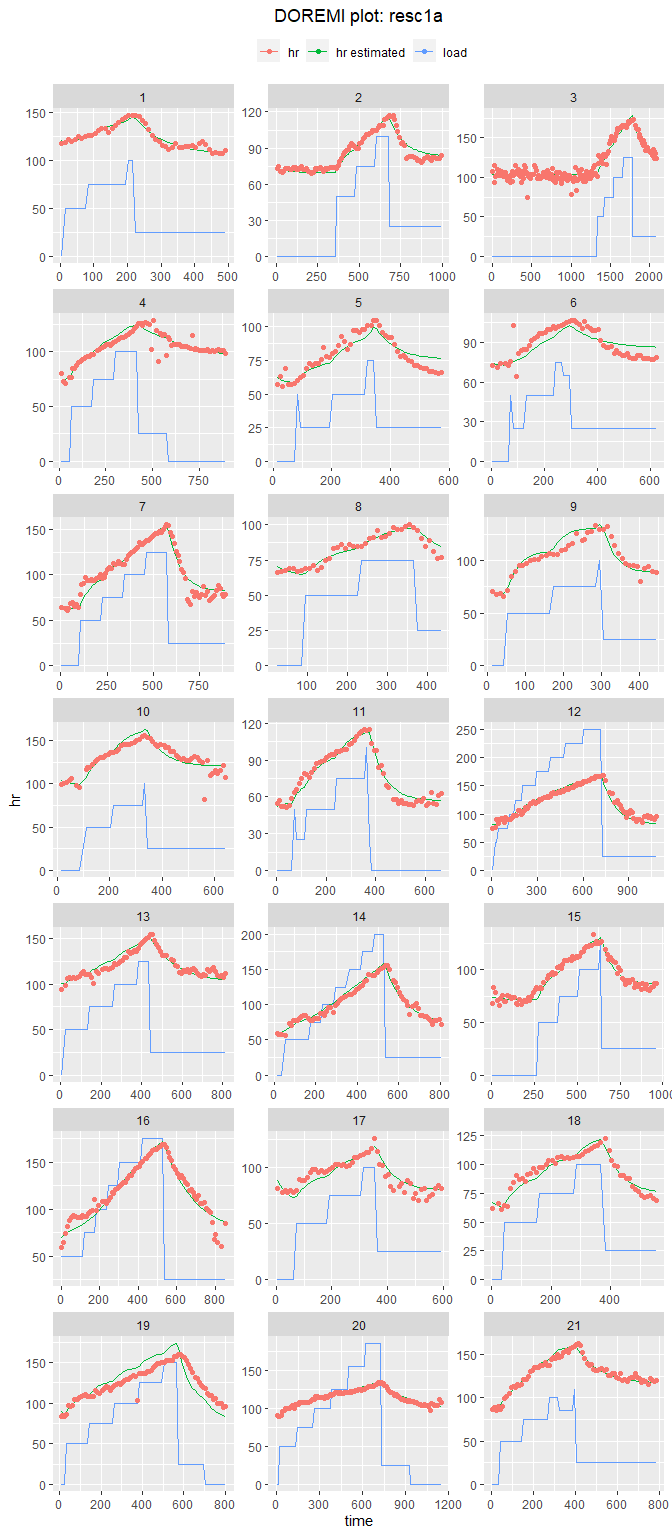As can be seen, the model reproduces reasonably well the variation of the heart rate, and can be used to fully characterize the heart rate dynamics with three simple parameters: the resting heart rate (the equilibrium value), the characteristic time of heart rate change (the decay time), and the increase of heart rate for a given effort (the gain).

Such analysis has been used in (Mongin et al., 2020a) and Mongin et al., 2020b.

## Measuring heart frequency during effort tests by considering several excitations

In the previous example, each one of the steps in the resistance load can be considered as a separated perturbation having an effect on heart rate. In this case, the functions will estimate different gains as explained, to account for each one of the contributions of the load to the total heart rate dynamics. Let us first create a new column for each step of effort load:

mydata <- cardio[id %in% 1:10]
# create a index indicate which step of the exercise test
# transforming to large format, to have one column per workload step
mydata_large <- data.table::dcast(id + time + hr  ~ paste0("load_",load_idx),data = mydata,value.var = "load")
# replacing NAs by 0s
load_cols <- paste0("load_",1:max(mydata$load_idx)) mydata_large[,c(load_cols) := lapply(.SD,function(col) data.table::fifelse(is.na(col),0,col)),.SDcols = load_cols] head(mydata_large) #> id time hr load_1 load_2 load_3 load_4 load_5 load_6 load_7 #> 1: 1 4 118 0 0 0 0 0 0 0 #> 2: 1 14 119 0 50 0 0 0 0 0 #> 3: 1 24 122 0 50 0 0 0 0 0 #> 4: 1 34 120 0 50 0 0 0 0 0 #> 5: 1 44 122 0 50 0 0 0 0 0 #> 6: 1 54 125 0 50 0 0 0 0 0 To consider each effort step as an independent excitation associated with its own gain, we can use analyze.1order and give the vector of the excitation column as id argument: # analyzing resc1b <- analyze.1order(data = mydata_large, id = "id", input = load_cols, time = "time", signal = "hr", dermethod = "gold", derparam = 5) #> WARN [2021-01-19 14:28:22] Excitation signal load_2 is constant and is removed. Here we will estimate a gain per excitation column resc1b #> id gamma gamma_stde yeqgamma yeqgamma_stde tau yeq #> 1: All 0.007693634 0.002857514 0.6122927 0.2212309 129.9776 79.58433 #> load_2_kgamma load_2_kgamma_stde load_2_k load_3_kgamma load_3_kgamma_stde #> 1: 0.006661281 0.001369214 0.8658172 0.005590196 0.001688833 #> load_3_k load_4_kgamma load_4_kgamma_stde load_4_k load_5_kgamma #> 1: 0.7266002 0.006671328 0.003565423 0.8671231 0.003535968 #> load_5_kgamma_stde load_5_k load_6_kgamma load_6_kgamma_stde load_6_k #> 1: 0.001867571 0.4595966 0.0004774536 0.002649282 0.06205827 #> load_7_kgamma load_7_kgamma_stde load_7_k R2 #> 1: -0.003151768 0.1569559 -0.4096592 0.9575932 In the plot, each workload step of the exercise test appear in one different column. plot(resc1b,id=1:10)+ ggplot2::facet_wrap(~id,ncol=3,scales="free")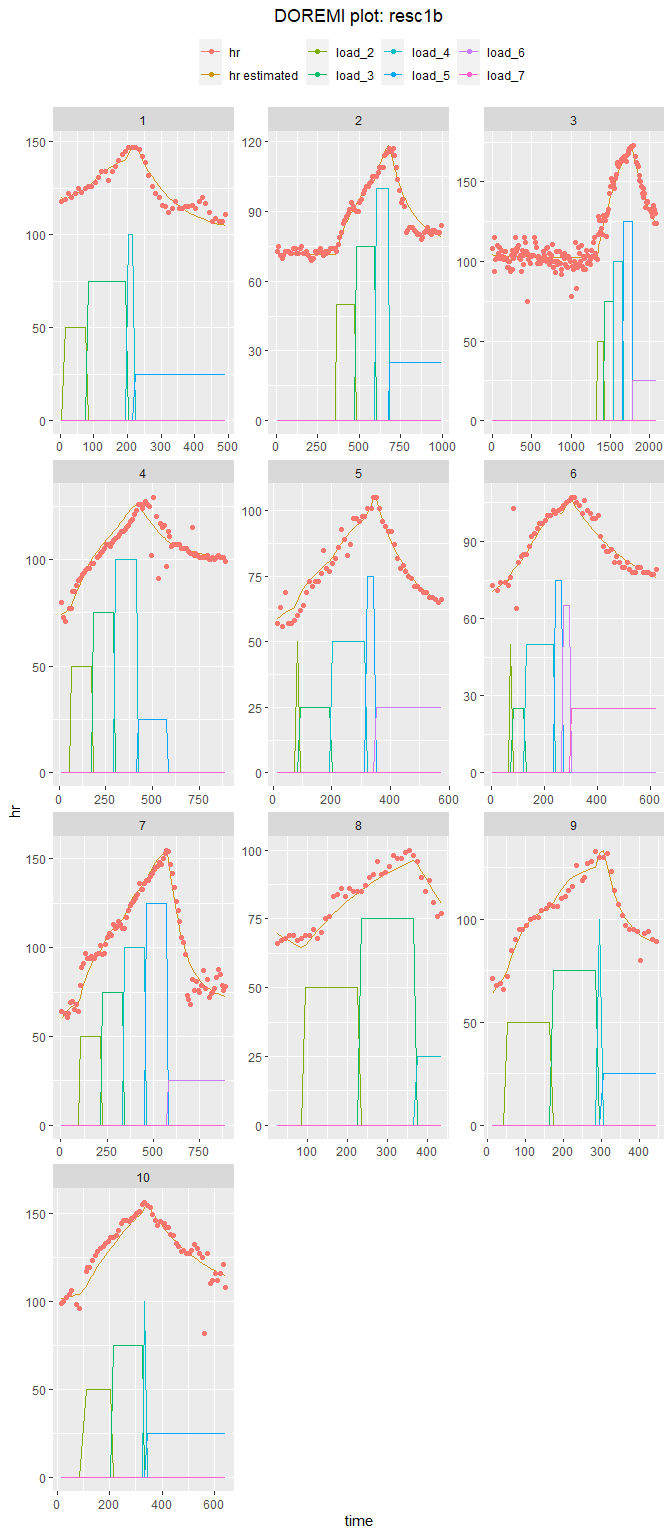This analysis has been the main analysis in Mongin et al.,2020c and used in Mongin et al.,2020d. ## Measurements of response time of individuals when carrying out mental rotation tasks Using the rotation dataset present in this package, we will apply the model to a case in which the excitation signal is not clearly defined. In brief, the data is from 17 individuals that carried out mental rotation tasks (identify if two figures, one of which is rotated, are the same or a mirror image) and the response time (in milliseconds) was measured (Courvoisier et al., 2013). Each individual was measured every day for 60 days, though there can be missing data. To account for this, the time has been represented by the number of days since the beginning of the experiment (variable days). The signal is the mean response time (variable meanRT) over the ~200 stimuli done every day. The purpose of the study was to compare the performance between men and women. dermethod<- "fda" pmin = 0.1 pmax = 1 pstep = 0.1 restemp <- optimum_param(data = rotation, id = "id", time ="days", signal = "meanRT", dermethod = dermethod, model = "1order", pmin = pmin, pmax = pmax, pstep = pstep) #> WARN [2021-01-19 14:28:31] No excitation signal introduced as input. Input was set to 0. #> WARN [2021-01-19 14:28:31] Some of the decay times calculated were negative and thus, the estimated signal was not generated for these. #> decay times can be negative for some individuals for the following reasons: 1. The signal of #> the individual doesn't go back to equilibrium. 2.The linear mixed-effects model estimating the random #> effect showed some error messages/warnings. 3.Model misspecification. #> WARN [2021-01-19 14:28:31] No excitation signal introduced as input. Input was set to 0. #> WARN [2021-01-19 14:28:31] Some of the decay times calculated were negative and thus, the estimated signal was not generated for these. #> decay times can be negative for some individuals for the following reasons: 1. The signal of #> the individual doesn't go back to equilibrium. 2.The linear mixed-effects model estimating the random #> effect showed some error messages/warnings. 3.Model misspecification. #> WARN [2021-01-19 14:28:31] No excitation signal introduced as input. Input was set to 0. #> WARN [2021-01-19 14:28:31] Some of the decay times calculated were negative and thus, the estimated signal was not generated for these. #> decay times can be negative for some individuals for the following reasons: 1. The signal of #> the individual doesn't go back to equilibrium. 2.The linear mixed-effects model estimating the random #> effect showed some error messages/warnings. 3.Model misspecification. #> WARN [2021-01-19 14:28:31] No excitation signal introduced as input. Input was set to 0. #> WARN [2021-01-19 14:28:32] Some of the decay times calculated were negative and thus, the estimated signal was not generated for these. #> decay times can be negative for some individuals for the following reasons: 1. The signal of #> the individual doesn't go back to equilibrium. 2.The linear mixed-effects model estimating the random #> effect showed some error messages/warnings. 3.Model misspecification. #> WARN [2021-01-19 14:28:32] No excitation signal introduced as input. Input was set to 0. #> WARN [2021-01-19 14:28:32] No excitation signal introduced as input. Input was set to 0. #> WARN [2021-01-19 14:28:32] No excitation signal introduced as input. Input was set to 0. #> WARN [2021-01-19 14:28:32] No excitation signal introduced as input. Input was set to 0. #> WARN [2021-01-19 14:28:33] No excitation signal introduced as input. Input was set to 0. #> WARN [2021-01-19 14:28:33] No excitation signal introduced as input. Input was set to 0. restemp$summary_opt
resc2a <- analyze.1order(data = rotation,
id = "id",
time ="days",
signal = "meanRT",
dermethod = dermethod,
derparam = restemp\$d)
#> WARN [2021-01-19 14:28:33] No excitation signal introduced as input. Input was set to 0.

Plotting analysis results:

plot(resc2a, id = 1:17)